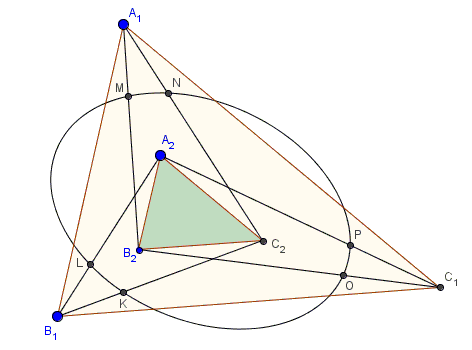# Two Similar Triangles with Parallel Sides

### Problem

Let there be two similar triangles $\Delta A_{1}B_{1}C_{1}$ and $\Delta A_{2}B_{2}C_{2}$ with the corresponding sides parallel: $A_{1}B_{1}\parallel A_{2}B_{2},$ etc. Let $r$ be a real number. Define six points $rA_{1}+(1-r)B_{2},$ $rA_{1}+(1-r)C_{2},$ $rB_{1}+(1-r)A_{2},$ $rB_{1}+(1-r)C_{2},$ $rC_{1}+(1-r)A_{2},$ and $rC_{1}+(1-r)B_{2}.$Prove that the six points lie on a conic.

### Hint

Pay attention to what kind of hexagon is formed by the six points.

### Solution

The opposite sides of the hexagon so obtained are parallel:Thus, we are in a position to apply the statement about a hexagon formed by three pairs of parallel lines: the six points are conconical. However, unlike in the general case, in the current problem the conic is always bounded, making it an ellipse.

### Acknowledgment

Dao Thanh Oai has posted the problem with $r=.5$ at the CutTheKnotMath facebook page, pointing out the difference with the general case.